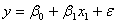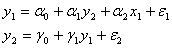# Introductory Econometrics

### Chapter 24: Simultaneous Equations

Throughout this book, we have used regression analysis in a variety of ways. From the simplest bivariate regression to consideration of the effects of heteroskedasticity or autocorrelation, we have always worked with a single equation. This chapter introduces you to simultaneous equations models (SEM). As the name makes clear, the heart of this class of models lies in a data generation process that depends on more than one equation interacting together to produce the observed data.

Unlike the single-equation model in which a dependent (y) variable is a function of independent (x) variables, other y variables are among the independent variables in each SEM equation. The y variables in the system are jointly (or simultaneously) determined by the equations in the system.

Compare the usual single equation DGP,,

to a simple, two-equation SEM:.

Notice that the first equation in the system has a conventional x variable, but it also has a dependent variable (y2) on the right-hand side. Likewise, the second equation has a dependent variable (y1) as a right-hand side variable. In a simultaneous equations system, variables that appear only on the right-hand side of the equals sign are called exogenous variables. They are truly independent variables because they remain fixed. Variables that appear on the right-hand side and also have their own equations are referred to as endogenous variables. Unlike exogenous variables, endogenous variables change value as the simultaneous system of equations grinds out equilibrium solutions. They are endogenous variables because their values are determined within the system of equations.

A natural question to ask is, What happens if we just ignore the simultaneity? Suppose, for example, we are interested only in the effect of y1 on y2. Could we simply toss out the first equation and treat the second one as a standalone, single equation, using our usual ordinary least squares regression to estimate the coefficients? In fact, this is what most single-equation regressions actually do – they simply ignore the fact that many x variables are not truly exogenous, independent variables. Unfortunately, it turns out that closing your eyes to the other equations is not a good move: the single equation OLS estimator of ? 1 is biased. This important result, called simultaneity bias, occurs because y1 is correlated with e2, as we will show in section 24.3.

Fortunately, there are ways to consistently estimate the coefficients in the system. The most common approach is called the method of instrumental variables or IV. When several instrumental variables are available, they are combined via regression (the first stage) and then used in a second regression. This procedure is called two-stage least squares, 2SLS (or TSLS).

We cannot hope to cover this wide and complex area of econometrics completely in this introductory text, but we can convey the essentials of SEMs. As we have done with other topics, we will focus on fundamental concepts, using concrete examples to illustrate key points.

The next section introduces a simple example used throughout the chapter. Section 24.3 shows how OLS on a single equation pulled from a simultaneous system of equations is hopelessly flawed. With OLS out of the picture, we then turn to a demonstration of how IV estimation via 2SLS works.

Excel Workbooks